Next: About this document ... Up: lab_template Previous: lab_template

Subsections

# Applications of exponential and logarithmic functions

## Purpose

The purpose of this lab is to use Maple to study applications of exponential and logarithmic functions. These are used to model many types of growth and decay, for example bacterial growth and radiaoctive decay. This lab also describes applications of exponential and logarithmic functions for heating and cooling and to medicine dosage

## Background

### Exponential growth

The simple model for growth is exponential growth, where it is assumed that
is proportional to. That is,Separating the variables and integrating (see section 4.4 of the text), we haveso thatIn the case of exponential growth, we can drop the absolute value signs around, becausewill always be a positive quantity. Solving for, we obtain
which we may write in the form
, whereis an arbitrary positive constant.

### Exponential Decay

In a sample of a radioactive material, the rate at which atoms decay is proportional to the amount of material present. That is,
whereis a constant. This is the same equation as in exponential growth, except thatreplaces
. The solution is
where
is a positive constant. Physically,is the amount of material present at
.

Radioactivity is often expressed in terms of an element's half-life. For example, the half-life of carbon-14 is 5730 years. This statement means that for any given sample of, after 5730 years, half of it will have undergone decay. So, if the half-life is of an element Z is
years, it must be that
, so that
and.

### Newton's law of cooling

What is usually called Newton's law of cooling is a simple model for the change in temperature of an object that is in contact with an environment at a different temperature. It says that the rate of change of the temperature of an object is proportional to the difference between the object's temperature and the temperature of the environment. Mathematically, this can be expressed as the differential equation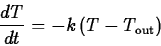whereis the constant of proportionality and
is the temperature of the environment. Using a technique called separation of variables it isn't hard to derive the solution
where
is the temperature of the object at.

### Effective medicine dosage

If a drug is administered to a patient intravenously, the concentration jumps to its highest level almost immediately. The concentration subsequently decays exponentially. If we use
to represent the concentration at time t, and
to represent the concentration just after the dose is administered then our exponential decay model would be given by
A problem facing physicians is the fact that for most drugs, there is a concentration,
, below which the drug is ineffective and a concentration,, above which the drug is dangerous. Thus the physician would like the have the concentrationsatisfy
This means that the initial dose must not produce a concentration larger thanand that another dose will have to be administered before the concentration reaches.

## Maple commands

The main functions you need are the natural exponential and natural logarithm. The Maple commands for these functions are exp and ln. Here are a few examples.
> f := x -> exp(-2*x);
> simplify(ln(3)+ln(9));
> ln(exp(x));
> simplify(ln(exp(x)),assume=real);

The assume=real is needed in the command above, because Maple usually works with complex variables. The command for base 10 logarithms is log10. Here are some examples. Note how Maple likes to convert base 10 logarithms to natural logarithms.
> log10(100);
> simplify(log10(100));
> simplify(log10(a)-log10(b),assume=real);


Sometimes you need to use experimental data to determine the value of constants in models. For example, suppose that for a particular drug, the following data were obtained. Just after the drug is injected, the concentration is 1.5 mg/ml (milligrams per milliliter). After four hours the concentration has dropped to 0.25 mg/ml. From this data we can determine values ofandas follows. The value ofis the initial concentration, so we have
To find the value ofwe need to solve the equation
which we get by plugging in
and using the data
. Maple commands for solving forand defining and plotting the functionare shown below.
> k1 := solve(0.25=1.5*exp(-4*k),k);
> C1 := t -> 1.5*exp(-k1*t);
> plot(C1(t),t=0..6);


## Exercises

1. In 1935 Charles F. Richter of Cal Tech developed a scale for measuring the magnitude of earthquakes. The Richter Scale formula is given by
whereis the magnitude of the earthquake,
is the amplitude of the largest seismic wave as measured on a standard seismograph 100 kilometers from the epicenter andis the amplitude of a reference earthquake of amplitude 1 micron on a standard seismograph at the same distance from the epicenter.
1. When the amplitude of an earthquake is tripled, by how much does the magnitude increase?
2. In 1989, the San Francisco Bay area suffered severe damage from an earthquake of magnitude 7.1. However, the damage was not nearly as extensive as that caused by the Alaska earthquake of 1964, which had magnitude 8.6. What is the ratio of the amplitude of the 1964 quake to the 1989 quake?

3. The 1906 San Francisco earthquake had an intensity 16 times that of the 1989 quake. What was the Richter scale magnitude of the 1906 quake?

2. A metal plate is heated to a temperature of
then allowed to cool in a room where the temperature is kept at. The plate cools so that the difference between the plate temperature and the room temperature decreases by half of the difference every 1/4 hour. What is the temperature of the plate after 1/4 hour? After 1/2 hour? After 1 hour?

3. Suppose that for a certain drug, the following results were obtained. Immediately after the drug was administered, the concentration was 8 mg/ml. Four hours later, the concentration had dropped to 2.3 mg/ml. Determine the value offor this drug.Next: About this document ... Up: lab_template Previous: lab_template
William W. Farr
2002-12-03